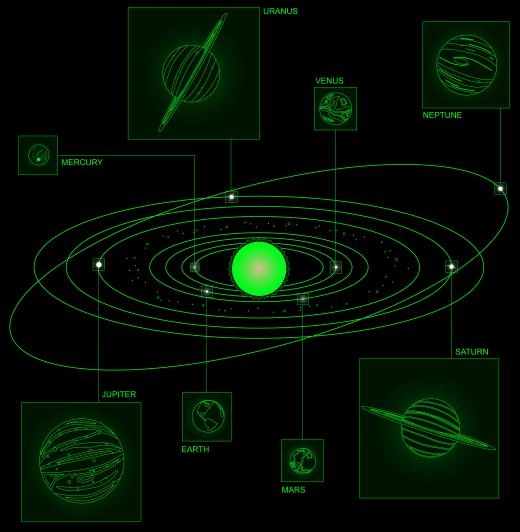Science
Fact Checked

# What is an Elliptic Orbit?

Amy Dyslex
Amy Dyslex

An elliptic orbit is the movement of one body around another in an oval-shaped path. It can be defined as a Kepler orbit that has an eccentricity ranging anywhere between 0 and 1. In the study of celestial mechanics, a Kepler orbit deals with the laws and principles of orbiting bodies moving in the form of an ellipse, hyperbola, or parabola. In astrodynamics, the eccentricity, sometimes called first eccentricity, of an orbit is a parameter that describes its actual shape and stretch.

Under the standard assumptions and principles of astrodynamics, an orbit must have a conic section shape. The conic eccentricity is then a numerical value. This number stands for a specific projection angle, defining the flatness or roundness of an elliptic orbit.All of the planets in the Solar System have elliptical orbits, though their eccentricity varies.

Eccentricity for an elliptic orbit can also be defined as a measure of deviation of an orbit from the shape of a standard circle. Orbital eccentricity for a perfect circular orbit is 0. This value serves as a benchmark to evaluate the divergence of any given elliptic orbit from the standard circle.

Earth's solar system is made up of numerous natural satellites like planets, moons, comets, and similar revolving bodies. These bodies orbit around their respective primaries — such as the Earth orbiting the Sun — along a fixed elliptic orbit. A general overview of their motion seems to give an impression of circular orbits. In reality, however, all heavenly bodies strictly follow elliptical orbits, with varying degrees of eccentric measures. The higher the value of eccentricity, the flatter and more elongated the shape of the elliptic orbit will be.

The eccentricity of the Earth’s elliptical orbit is at present measured to be 0.0167. This low value makes the elliptic orbit of earth almost a perfect circle. On the other hand, eccentric values for comets are close to 1, making their orbits nearly flat and elongated. In case of a gravitational two-body problem, an eccentricity measure between 0 and 1 allows both bodies to revolve in identical orbits. Popular examples of elliptic orbits are the Hohmann transfer orbit, the Molniya orbit, and the tundra orbit.

The concept of elliptic orbits was first discovered and promoted by Johannes Kepler, a German scientist in the early 17th century. The findings were published in his First Law of Planetary Motion, and brought forward important laws related to orbits of celestial bodies. These findings have aided scientists in understanding and studying the properties of an elliptic orbit.

The specific orbital energy, also known as vis-viva energy, is defined as the sum of potential energy and kinetic energy of an orbiting object. For an elliptic orbit, the specific energy is negative and is calculated irrespective of its eccentricity. The elliptical nature of planetary orbits is an important feature which determines seasonal changes, temperature zones, and the climatic zones of the respective planets.

## You might also Like